Next: Exercises Up: Lagrangian Dynamics Previous: Generalized Momenta

Spherical Pendulum

Consider a pendulum consisting of a compact mass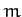on the end of light inextensible string of length. Suppose that the mass is free to move in any direction (as long as the string remains taut). Let the fixed end of the string be located at the origin of our coordinate system. We can define Cartesian coordinates, (,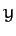,), such that the-axis points vertically upward. We can also define spherical coordinates, (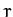,,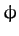), whose axis points along the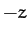-axis. The latter coordinates are the most convenient, sinceis constrained to always take the value. However, the two angular coordinates,and, are free to vary independently. Hence, this is a two degree of freedom system.

The Cartesian coordinates can be written in terms of the angular coordinatesand. In fact,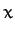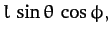(657)(658)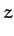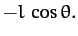(659)

Hence, the potential energy of the system is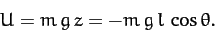(660)

Also,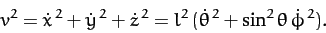(661)

Thus, the Lagrangian of the system is written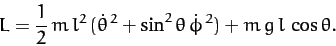(662)

Note that the Lagrangian is independent of the angular coordinate. It follows that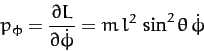(663)

is a constant of the motion. Of course,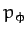is the angular momentum of the system about the-axis. This is conserved because neither the tension in the string nor the force of gravity exert a torque about the-axis. Conservation of angular momentum about the-axis implies that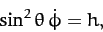(664)

where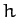is a constant.

The equation of motion of the system,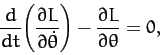(665)

yields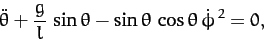(666)

or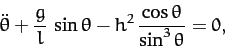(667)

where use has been made of Equation (664).

Suppose that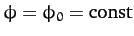. It follows that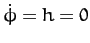. Hence, Equation (667) yields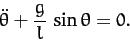(668)

This, of course, is the equation of a simple pendulum whose motion is restricted to the vertical plane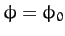--see Section 3.10.

Suppose that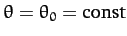. It follows from Equation (664) that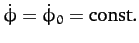: i.e., the pendulum bob rotates uniformly in a horizontal plane. According to Equations (664) and (667),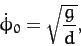(669)

where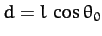is the vertical distance of the plane of rotation below the pivot point. This type of pendulum is usually called a conical pendulum, since the string attached to the pendulum bob sweeps out a cone as the bob rotates.

Suppose, finally, that the motion is almost conical: i.e., the value ofremains close to the value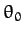. Let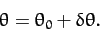(670)

Taylor expanding Equation (667) to first order in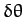, the zeroth order terms cancel out, and we are left with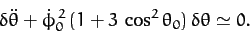(671)

Hence, solving the above equation, we obtain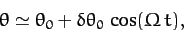(672)

where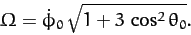(673)

Thus, the angleexecutes simple harmonic motion about its mean valueat the angular frequency.

Now the azimuthal angle,, increases by(674)

as the angle of inclination to the vertical,, goes between successive maxima and minima. Suppose thatis small. In this case,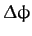is slightly greater than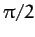. Now ifwere exactlythen the pendulum bob would trace out the outline of a slightly warped circle: i.e., something like the outline of a potato chip or a saddle. The fact thatis slightly greater thanmeans that this shape precesses about the-axis in the same direction as the direction rotation of the bob. The precession rate increases as the angle of inclinationincreases. Suppose, now, thatis slightly less than. (Of course,can never exceed). In this case,is slightly less than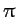. Now ifwere exactlythen the pendulum bob would trace out the outline of a slightly tilted circle. The fact thatis slightly less thanmeans that this shape precesses about the-axis in the opposite direction to the direction of rotation of the bob. The precession rate increases as the angle of inclinationdecreases below.Next: Exercises Up: Lagrangian Dynamics Previous: Generalized Momenta
Richard Fitzpatrick 2011-03-31